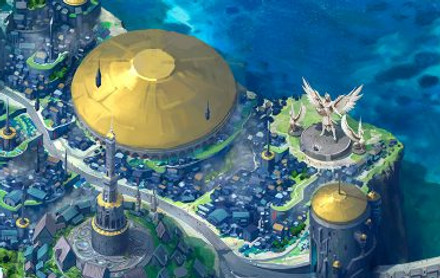top of page

### The quests are listed in order of the story. They are designed for standard mastery so each quest covers different topics under each standard. To follow the story, the quests must be completed in order.7.N.1 Read, write, represent, and compare rational numbers, expressed as integers, fractions, and decimals.

7.N.2 Calculate with integers and rational numbers, with and without positive integer exponents, to solve realworld and mathematical problems; explain the relationship between absolute value of a rational number and the distance of that number from zero.

7.A.1 Understand the concept of proportionality in real-world and mathematical situations, and distinguish between proportional and other relationships.

7.A.2 Recognize proportional relationships in real-world and mathematical situations; represent these and other relationships with tables, verbal descriptions, symbols, and graphs; solve problems involving proportional relationships and interpret results in the original context.

7.A.3 Represent and solve linear equations and inequalities.

7.A.4 Use order of operations and properties of operations to generate equivalent numerical and algebraic expressions containing rational numbers and grouping symbols; evaluate such expressions.

7.GM.1 Develop and understand the concept of surface area and volume of rectangular prisms.

7.GM.2 Determine the area of trapezoids and area and perimeter of composite figures.

7.GM.3 Use reasoning with proportions and ratios to determine measurements, justify formulas, and solve real-world and mathematical problems involving circles and related geometric figures.

7.GM.4 Analyze the effect of dilations, translations, and reflections on the attributes of two-dimensional figures on and off the coordinate plane.

7.D.1 Display and analyze data in a variety of ways.

7.D.2 Calculate probabilities and reason about probabilities using proportions to solve real-world and mathematical problems.

bottom of page